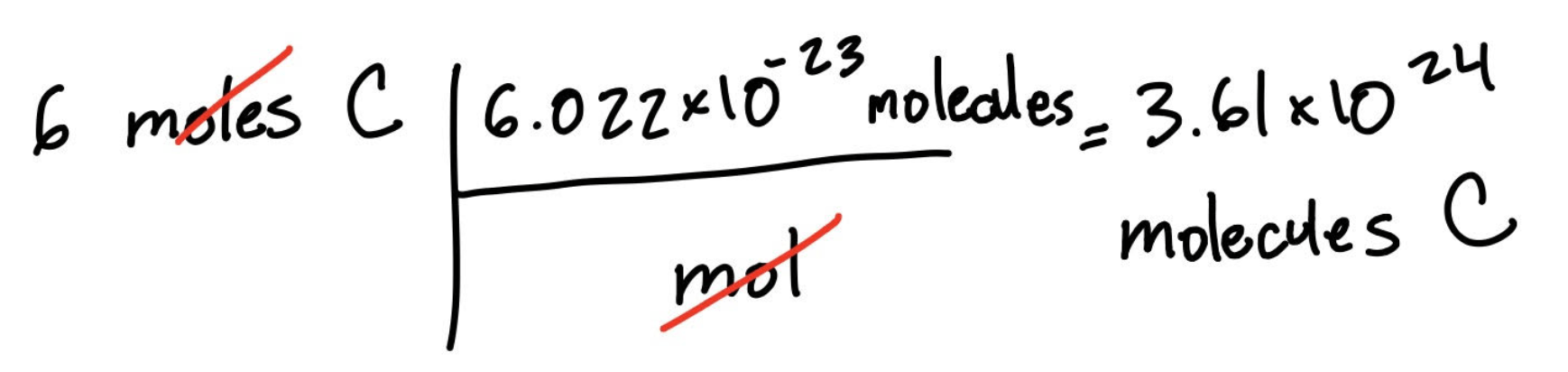Cyber Monday Sale: ALL MCAT Products & Admissions Services

MCAT Content / Stoichiometry / Mole Concept Avogadros Number Na

### Mole concept, Avogadro’s number NA

Topic: Stoichiometry

A mole is a unit in chemistry that defines the number of elemental entities in a certain mass of a given substance and is represented by Avogadro’s number, which is 6.022×1023.

The mol (plural: moles), also referred to as Avogadro’s number (NA), is 6.022×1023 elementary entities (which can be defined as atoms, molecules, ions, or electrons) per one mol. Because Avogadro’s number will always be some entity over one mol, we can write it as:For example, if we wanted to know how many atoms there are in 6 moles of carbon, we could set up the following:Likewise, if we wanted to know the number of molecules in 6 moles of carbon, we could set up an equation with the same number we used for converting to atoms, but with molecules instead of atoms:Avogadro’s number is an SI unit and can be used with other SI units such as grams (g), and liters (L).

Practice Questions

MCAT Official Prep (AAMC)

Chemistry Question Pack Passage 3 Question 10

Key Point

• One mol is defined by Avogadro’s number, which is 6.022×1023 atoms/molecules/ions/electrons per mol; plural of mol is moles.

• Avogadro’s number is often used in chemistry to convert mass to a number of particles.

Key Terms

atom: the most fundamental and smallest part of an element (such as hydrogen) that can exist.

molecule: two or more atoms bonded together

SI units: International System of Units is the modern form of the metric system

avogadros number: the number of atoms in one mole of substance (6.02×1023)

Billing Information